Over a million developers have joined DZone.{{announcement.body}}
{{announcement.title}}

# Classification From Scratch, Part 9 of 8: Trees

DZone 's Guide to

# Classification From Scratch, Part 9 of 8: Trees

### In this post, we examine decision trees and the heuristics of the algorithms inside these tress that make them valuable in big data.

· Big Data Zone ·
Free Resource

Comment (0)

Save
{{ articles.views | formatCount}} Views

This is the ninth post of our series on classification from scratch. Today, we’ll see the heuristics of the algorithm inside classification trees. And yes, I promised eight posts in that series, but clearly, that was not sufficient… sorry for the poor prediction.

## Decision Tree

Decision trees are easy to read. So easy to read that they are everywhere:We start from the top, and we go down, with a binary choice, at each stop, each node. Let us see how it works on our dataset.

library(rpart)
cart = rpart(PRONO~.,data=myocarde)
library(rpart.plot)
prp(cart,type=2,extra=1)We start here with one single leaf. If we have two explanatory variables (the x-axis and the y-axis, if we want to plot it), we will check what happens if we cut the leaf according to the value of the first variable (and there will be two subgroups, the one on the left and the one on the right)Or if we cut according to the second one (and there will be two subgroups, the one on top and the one below).Why and where do we cut? Let us formalize this a little bit. A node (a leaf) constains observations, i.e. {yi,x)i}) for some I⊂{1,⋯,n}. Hence, a leaf characterized by I. For instance, the first node in the tree is I={1,⋯,n}. A (binary) split is based on one specific variable – say xj – and a cutoff, say s. Then, there are two options:

• either xi,js, then observation i goes on the left, in IL
• or xi,j>s, then observation i goes on the right, in IR

Thus, I =ILIR.

Now, define some impurity index, in some nodes. In the context of a classification tree, the most popular index used (the so-called impurity index) is Gini for node I is defined as: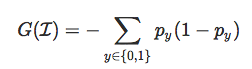where py is the proportion of individuals in the leaf of type y. I use this notation here because it can be extended to the case of more than one class. Here, we consider only binary classification. Now, why py(1−py)? Because we want leaves that are extremely homogeneous. In our dataset, out of 71 individuals, 42 died, 29 survived. A perfect classification would be obtained if we can split in two, with the 29 survivors on the left, and the 42 dead on the right. In that case, leaves would be perfectly homogeneous. So, when p0≈1 or p1≈1, we have strong homogeneity. If we want an index to maximize, py(1−py) might be an interesting candidate. Furthermore, the worst case would be a leaf with p0≈1/2, which is exactly what we have here. Note that we can also write: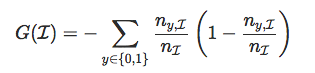where ny,I is the number of individuals of type y in the leaf I, and nI is the number of individuals in the leaf I.

If we do not split, we have index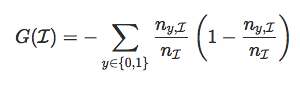while if we split, define index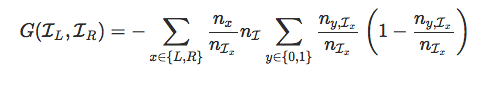The code to compute this would be:

gini = function(y,classe){
T. = table(y,classe)
nx = apply(T,2,sum)
n. = sum(T)
pxy = T/matrix(rep(nx,each=2),nrow=2)
omega = matrix(rep(nx,each=2),nrow=2)/n
g. = -sum(omega*pxy*(1-pxy))
return(g)}

Actually, one can consider other indices, like the entropic measure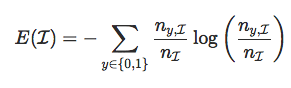while if we split,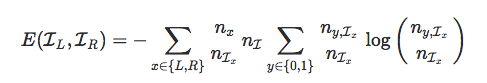entropy = function(y,classe){
T. = table(y,classe)
nx = apply(T,2,sum)
n. = sum(T)
pxy = T/matrix(rep(nx,each=2),nrow=2)
omega = matrix(rep(nx,each=2),nrow=2)/n
g  = sum(omega*pxy*log(pxy))
return(g)}

This index was used originally in the C4.5 algorithm.

## Dividing a Leaf (or Not)

For instance, consider the very first split. Assume that we want to split according to the very first variable

CLASSE = myocarde[,1] &lt;=100
table(CLASSE)
CLASSE
FALSE  TRUE
13    58

In that case, there will be 13 individuals on one side (the left, say), and 58 on the other side (the right).

gini(y=myocarde$PRONO,classe=CLASSE)  -0.4640415 Initially, without any split, it was -2*mean(myocarde$PRONO)*(1-mean(myocarde$PRONO))  -0.4832375 which can actually also be obtained with CLASSE = myocarde[,1] gini(y=myocarde$PRONO,classe=CLASSE)
 -0.4832375

There is a net gain in the splitting of the following:

gini(y=myocarde$PRONO,classe=(myocarde[,1]&lt;=100))- gini(y=myocarde$PRONO,classe=(myocarde[,1]&lt;=Inf))
 0.01919591

Now, how do we split? Which variable and which cutoff? Well… let’s try all possible splits. Here, we have 7 variables. We can consider all possible values, using

sort(unique(myocarde[,1]))

But in massive datasets, it can be very long. Here, I prefer

seq(min(myocarde[,1]),max(myocarde[,1]),length=101)

so that we try 101 values as possible cutoffs. Overall, the number of computations is rather low, with 707 Gini indices to compute. Again, I won’t get back here on the motivations for such a technique to create partitions, I will keep that for the course in Barcelona, but it is fast.

mat_gini = mat_v=matrix(NA,7,101)
for(v in 1:7){
variable=myocarde[,v]
v_seuil=seq(quantile(myocarde[,v],
6/length(myocarde[,v])),
quantile(myocarde[,v],1-6/length(
myocarde[,v])),length=101)
mat_v[v,]=v_seuil
for(i in 1:101){
CLASSE=variable&lt;=v_seuil[i]
mat_gini[v,i]=
gini(y=myocarde$PRONO,classe=CLASSE)}} Actually, the range of possible values is slightly different: I do not want to cutoff too much on the left or on the right… having a leaf with one or two observations is not the idea, here. Now, if we plot all the functions, we get par(mfrow=c(3,2)) for(v in 2:7){ plot(mat_v[v,],mat_gini[v,],type="l", ylim=range(mat_gini),xlab="",ylab="", main=names(myocarde)[v]) abline(h=max(mat_gini),col="blue") }Here, the most homogenous leaves obtained using a cut in two parts is when we use the variable ‘INSYS.’ And the optimal cutoff variable is close to 19. So far, that’s the only information we use. Well, actually no. If the gain is sufficiently large, we go for a split. Here, the gain is gini(y=myocarde$PRONO,classe=(myocarde[,3]&lt;19))-
gini(y=myocarde$PRONO,classe=(myocarde[,3]&lt;=Inf))  0.2832801 This is large. Sufficiently large to go for it, and to split it in two. Actually, we look at the relative gain -(gini(y=myocarde$PRONO,classe=(myocarde[,3]&lt;19))-
gini(y=myocarde$PRONO,classe=(myocarde[,3]&lt;=Inf)))/ gini(y=myocarde$PRONO,classe=(myocarde[,3]&lt;=Inf))
 0.5862131

If that gain exceeds 1% (the default value in R), we split it in two.

Then, we do it again. Twice. First, on the leaf on the left, with 27 observations. And we try to see if we can split it.

idx = which(myocarde$INSYS&lt;19) mat_gini = mat_v = matrix(NA,7,101) for(v in 1:7){ variable = myocarde[idx,v] v_seuil = seq(quantile(myocarde[idx,v], 7/length(myocarde[idx,v])), quantile(myocarde[idx,v],1-7/length( myocarde[idx,v])), length=101) mat_v[v,] = v_seuil for(i in 1:101){ CLASSE = variable&lt;=v_seuil[i] mat_gini[v,i]= gini(y=myocarde$PRONO[idx],classe=CLASSE)}}
par(mfrow=c(3,2))
for(v in 2:7){
plot(mat_v[v,],mat_gini[v,],type="l",
ylim=range(mat_gini),xlab="",ylab="",
main=names(myocarde)[v])
abline(h=max(mat_gini),col="blue")
}

The graph is here the following:Observe that the best split is obtained using ‘REPUL,’ with a cutoff around 1585. We check that the (relative) gain is sufficiently large, and then we go for it.

And then, we consider the other leaf, and we run the same code:

idx = which(myocarde$INSYS&gt;=19) mat_gini = mat_v = matrix(NA,7,101) for(v in 1:7){ variable=myocarde[idx,v] v_seuil=seq(quantile(myocarde[idx,v], 6/length(myocarde[idx,v])), quantile(myocarde[idx,v],1-6/length( myocarde[idx,v])), length=101) mat_v[v,]=v_seuil for(i in 1:101){ CLASSE=variable&lt;=v_seuil[i] mat_gini[v,i]= gini(y=myocarde$PRONO[idx],
classe=CLASSE)}}
par(mfrow=c(3,2))
for(v in 2:7){
plot(mat_v[v,],mat_gini[v,],type="l",
ylim=range(mat_gini),xlab="",ylab="",
main=names(myocarde)[v])
abline(h=max(mat_gini),col="blue")
}Here, we should split according to ‘REPUL,’ and the cutoff is about 1094. Here again, we have to make sure that the split is worth it. And we cut.

Now we have four leaves. And we should run the same code, again. Actually, not on the very first one, which is homogenous. But we should do the same for the other three. If we do it, we can see that we cannot split them any further. Gains will not be sufficiently interesting.

Now guess what… that’s exactly what we have obtained with our initial codeNote that the case of categorical explanatory variables has been discussed in a previous post, a few years ago.

## Application on Our Small Dataset

On our small dataset, we obtain (after changing the default values since,in R, we should not have leaves with less than 10 observations… and here, the dataset is too small).

tree = rpart(y ~ x1+x2,data=df,
control = rpart.control(cp = 0.25,
minsplit = 7))
prp(tree,type=2,extra=1)u = seq(0,1,length=101)
p = function(x,y){predict(tree,newdata=data.frame(x1=x,x2=y),type="prob")[,2]}
v = outer(u,u,p)
image(u,u,v,xlab="Variable 1",ylab="Variable 2",col=clr10,breaks=(0:10)/10)
points(df$x1,df$x2,pch=19,cex=1.5,col="white")
points(df$x1,df$x2,pch=c(1,19)[1+z],cex=1.5)
contour(u,u,v,levels = .5,add=TRUE)

We have a nice and simple cutWith less observations in the leaves, we can easily get a perfect model here:

tree = rpart(y ~ x1+x2,data=df,
control = rpart.control(cp = 0.25,
minsplit = 2))
prp(tree,type=2,extra=1)u = seq(0,1,length=101)
p = function(x,y){predict(tree,newdata=data.frame(x1=x,x2=y),type="prob")[,2]}
v = outer(u,u,p)
image(u,u,v,xlab="Variable 1",ylab="Variable 2",col=clr10,breaks=(0:10)/10)
points(df$x1,df$x2,pch=19,cex=1.5,col="white")
points(df$x1,df$x2,pch=c(1,19)[1+z],cex=1.5)
contour(u,u,v,levels = .5,add=TRUE)Nice, isn’t it? Now, just two little additional comments before growing some more trees…

## Pruning

I did not mention pruning here. Because there are two possible strategies when growing trees. Either we keep splitting until we obtain only homogeneous leaves. Once we have a big, deep tree, we go for pruning. Or we use the following strategy: at each step, we check if the split is worth it. If not, we stop.

## Variable Importance

An interesting tool is the variable importance function. The heuristic idea is that if we use variable ‘INSYS’ to split, it is an important variable. And its importance is related to the gain in the Gini index. If we get back to the visualization of the tree, it seems that two variables are interesting here: ‘INSYS’ and ‘REPUL.’ And we should get back to the previous computation to quantify how important they both are.This will be used in our next post, on random forests. But, actually, it is not the case here, with one single tree. Let us get back to the graph on the initial node.Indeed, ‘INSYS’ is important, since we decided to use it. But what about ‘INCAR’ or ‘REPUL’? They were very close… And actually, in R, those surrogate splits are considered in the computation, as briefly explained in the vignette. Let us look more carefully at the output of the R function

cart = rpart(PRONO~., myocarde)
split = summary(cart)$splits If we look at the first part of that object, we get split count ncat improve index adj INSYS 71 -1 0.58621312 18.850 0.0000000 REPUL 71 1 0.55440034 1094.500 0.0000000 INCAR 71 -1 0.54257020 1.690 0.0000000 PRDIA 71 1 0.27284114 17.000 0.0000000 PAPUL 71 1 0.20466714 23.250 0.0000000 So indeed, ‘INSYS’ was the most important variable, but surrogate splits can also be considered, and ‘INCAR’ and ‘REPUL’ are indeed very important. The gain was 58% (as we obtained) using ‘INSYS’ but there were gains of 55% (nothing to be ashamed of). So it would be unfair to claim that they have no importance, at all. And it is the same for the other leaves that we split, REPUL 27 1 0.18181818 1585.000 0.0000000 PVENT 27 -1 0.10803571 14.500 0.0000000 PRDIA 27 1 0.10803571 18.500 0.0000000 PAPUL 27 1 0.10803571 22.500 0.0000000 INCAR 27 1 0.04705882 1.195 0.0000000 On the left, we did use ‘REPUL’ (with 18% gain), but ‘PVENT,’ ‘PRDIA,’ and ‘PAPUL’ were not that bad, with (almost) 11% gain. We can obtain variable importance by summing all those values, and we have cart$variable.importance
INSYS      REPUL      INCAR      PAPUL      PRDIA      FRCAR      PVENT
10.3649847 10.0510872  8.2121267  3.2441501  2.8276121  1.8623046  0.3373771

We can visualize this using:

barplot(t(cart\$variable.importance),horiz=TRUE)To be continued with more trees…

Topics:
big data ,r ,decision trees ,data nodes

Comment (0)

Save
{{ articles.views | formatCount}} Views

Published at DZone with permission of

Opinions expressed by DZone contributors are their own.